Dense Voxelization into a Sparsely Allocated 3D Lattice

Sparsely allocated (partially resident) textures are exposed through an OpenGL extension (GL_ARB_sparse_texture), allowing us to allocate virtual textures as a portion of the GPU’s virtual addressing space, and commit to physical memory only specific pages as needed.

This post aims to cover the voxelization process, using a sparse 3D image serving as a lattice for the voxels’ data structure.

# Why Dense Voxelization?

Dense voxelization trades memory for speed, and provides good random access capabilities, increasing voxelization and ray/cone marching performance. A sparsely allocated 3D image offsets some of the heavy memory requirements, allowing for fine voxel resolution on 2/4GB hardware.

# Overview

To be able to voxelize the entire scene in real-time, allowing for completely dynamic meshes and light sources, a very fast and simple data structure is needed. Unlike sparse Bounding Volume Hierarchys, a dense 3D image allows random access to voxels, and can be quickly upsampled into an octree via mipmapping.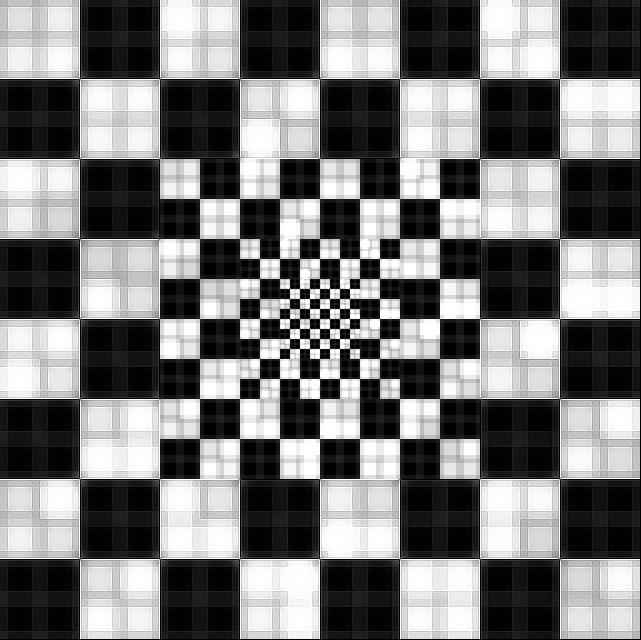A typical $1024^3$ 3D image of RGBA format with mipmaps would take way too much memory. However the further away from the camera we get, the lower the detail required and the less the size the voxels should be. To do this we commit only the center region of the level 0 to memory, likewise we commit a region of double world size of level 1 (but same amount of texels), etc..

The size of the image should be divisible by the number of mipmaps (or steps) times the page size.

As a minimum, we are interested in storing color and normal data per voxel. To do so we use 2 3D images of RGBA format, where the ‘w’ component of the normal texture is used as a counter for conflict resolution. We use 16-bit floating point textures to allow atomic operations using the GL_NV_shader_atomic_fp16_vector extension (we will discuss that later).

# The Voxelization Pipeline

I will provide an overview of the 6-plane separable voxelization process. We will use a technique similar to the one outlined in “Octree-Based Sparse Voxelization Using the GPU Hardware Rasterizer” in OpenGL Insights, and the conservative rasterization approach as described by Hasselgren et al. 05 in GPU Gems 2. Both are freely available online, and should be reviewed for additional details about the voxelization pipeline.

To voxelize, we turn off color and depth writes and draw the scene. The vertex program is as simple as it gets, and is only responsible for transforming positions and normals to world-space (multiplying by the model matrix, and the transpose of the inverse of the model matrix respectively) as well as passing additional vertex attributes to the geometry shader.

# Voxelization: The Geometry Shader

The grunt of the preparation work is done in the geometry shader. We want the rasterizer to generate a fragment for each voxel. To do so, first we scale by the approximated voxel size for the triangle. The voxel size can be estimated by calculating the distance to the triangle. A true point↔triangle distance is too expensive, instead we compute the distance d to the AABB of the triangle.

Let ($p_0, p_1, p_2$) be the input triangle’s gl_Positions to the geometry shader. Given $s = \mathrm{voxel\_size}(d)$:

\begin{aligned} & p_0 = p_0 / s \\ & p_1 = p_1 / s \\ & p_2 = p_2 / s \end{aligned}

To generate voxel fragments we project the triangle orthographically along the triangle’s most dominant axis-aligned face. In other words, we want to rotate the triangle so the normal “points towards us”. To do so the scaled vertices of the triangle $p_0, p_1, p_2$ need to be transformed to screen-space using a basis transform matrix $\mathbf{T}_{3\times 3}$. The matrix $\mathbf{T}$ is an orthonormal (rotation) matrix and we compute it using the triangle normal $n$. For the conservative rasterization process we also want the triangle to be front facing, therefore we rotate around the x or y axis, depending on the normal.

$$\mathbf{T}_{3\times 3} = \begin{cases} \begin{pmatrix} 0 & 0 & -\mathrm{sign}(n_x) \\ 0 & 1 & 0 \\ \mathrm{sign}(n_x) & 0 & 0 \end{pmatrix} &\;\text{if }|n_x\ge n_y|\ \text{and}\ |n_x\ge n_z| \\ \begin{pmatrix} 1 & 0 & 0 \\ 0 & 0 & -\mathrm{sign}(n_y) \\ 0 & \mathrm{sign}(n_y) & 0 \end{pmatrix}&\;\text{if }|n_y\ge n_x|\ \text{and}\ |n_y\ge n_z| \\ \begin{pmatrix} \mathrm{sign}(n_z) & 0 & 0 \\ 0 & 1 & 0 \\ 0 & 0 & \mathrm{sign}(n_z) \end{pmatrix}&\;\text{otherwise} \ \end{cases}$$

At this point we can transform the triangle and its normal to screen-space. (remember, $\mathbf{T}$ is an orthonormal matrix, therefore $\mathbf{T}^{-1} = \mathbf{T}^\intercal$)

\begin{aligned} & u = \mathbf{T}^\intercal p_0 \\ & v = \mathbf{T}^\intercal p_1 \\ & w = \mathbf{T}^\intercal p_2 \\ & n = \mathbf{T}^\intercal n \end{aligned}

# Geometry Shader: Conservative Rasterization

Assuming no multisampling (which reduces, but doesn’t solve, the problem), the hardware rasterizer generates fragments for pixels whose centers pass the triangle test. This means we will miss voxels.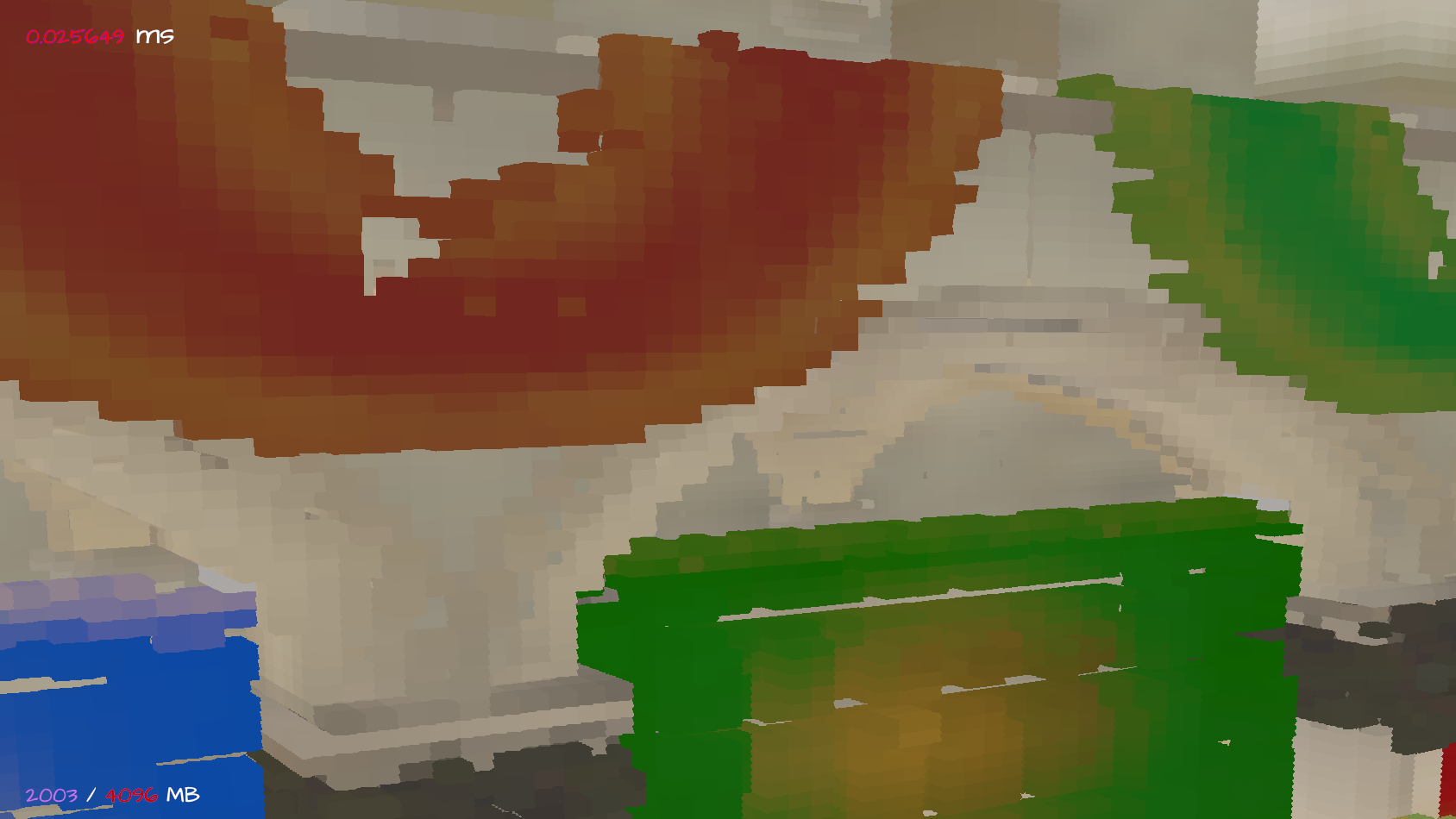Missing voxels due to fragments not passing the triangle test

To handle that a conservative rasterization process will be employed, i.e. the geometry shader should generate a bounding triangle, and the fragment program will have to filter excess fragments.

To filter fragments we need to calculate the axis-aligned bounding box.

aabb.start = min{ u.xy, v.xy, w.xy };
aabb.size = max{ u.xy, v.xy, w.xy } - aabb.start + vec2(1);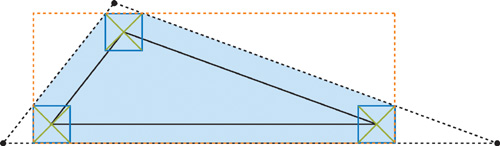Conservative rasterization: Bounding triangle (source: GPU Gems 2)

The bounding triangle ($q, r, s$) screen-space coordinates xy are estimated and then the z coordinate is calculated using the plane equation.

d = dot(u, n);
q.xy = bounding_triangle_vertex(w, u, v)
r.xy = bounding_triangle_vertex(u, v, w)
s.xy = bounding_triangle_vertex(v, w, u)
q.z = (d - dot(q.xy, n.xy)) / n.z
r.z = (d - dot(r.xy, n.xy)) / n.z
s.z = (d - dot(s.xy, n.xy)) / n.z


The method bounding_triangle_vertex takes 3 vertices, creates planes parallel to z that go through the edges, offsets them and computes their intersection vector. The planes are perpendicular to the xy plane, therefore the intersection is also perpendicular to the xy plane and the vector is projected to a point, i.e. the vertex of the bounding triangle.

The method assumes front-facing triangles and will generate opposite results for back faces, shrinking instead of expanding.

vec2 bounding_triangle_vertex(vec3 U, vec3 V, vec3 W) {
vec3 prev = vec3(U.xy, 1);
vec3 v = vec3(V.xy, 1);
vec3 next = vec3(W.xy, 1);

vec3 a = v - prev;
vec3 b = next - v;

vec3 p0 = cross(a, prev);
vec3 p1 = cross(b, v);

p0.z -= dot(vec2(.5f), abs(p0.xy));
p1.z -= dot(vec2(.5f), abs(p1.xy));

vec3 t = cross(p0, p1);
return t.xy / t.z;
}


Before emitting a primitive from the geometry shader, the vertices need to be transformed to clip-space and the vertex attributes calculated. As a bare minimum we need the stretched world positions of the vertices as vertex attributes. The hardware will interpolate the positions, giving us voxel coordinates per fragment. Texture coordinates and normals are usually passed as well.

To recover the modified vertices’ world-space coordinates we transform the stretched vertices from screen-space using the scale factor and the transformation matrix.

\begin{aligned} & \bar{u} = s\mathbf{T}q \\ & \bar{v} = s\mathbf{T}r \\ & \bar{w} = s\mathbf{T}s \end{aligned}

The clip space coordinates ($v_0, v_1, v_2$) are easily computed from the screen-space coordinates ($q, r, s$).

v0 = (q.xy - aabb.start) / (voxels_image_size >> 1) - vec2(1);
v1 = (r.xy - aabb.start) / (voxels_image_size >> 1) - vec2(1);
v2 = (s.xy - aabb.start) / (voxels_image_size >> 1) - vec2(1);


At this point we are ready to emit vertices and generate the primitive.

out.world_position = u_;
out.gl_Position = vec4(v0, 0, 1);
out.uv = in.uv;
out.world_position = v_;
out.gl_Position = vec4(v1, 0, 1);
out.uv = in.uv;
out.world_position = w_;
out.gl_Position = vec4(v2, 0, 1);
out.uv = in.uv;


# Early Voxelization in the Geometry Shader

For large triangles the approach outlined above makes efficient use of the rasterization pipeline of current hardware. However for triangles spanning less than a single voxel this can be wasteful. To handle small triangles an early voxelization approach can allow us to skip the fragment program entirely and save a lot of unnecessary computations.

This can be done early in the geometry shader: Check if the scaled vertices ($q, r, s$) round to the same integer coordinates. If so, the voxelization procedure can be executed in the geometry shader and no primitives are to be generated.

# Voxelization: The Fragment Shader

The fragment program is mainly responsible for filtering excess fragments and writing the voxels to the data structure, the sparse 3D image.

First we discard fragments that don’t pass the AABB test, i.e. any fragment for which gl_FragCoord > aabb.size.

Then we convert the voxel fragments into actual voxels. Note that the voxel → fragment correspondence isn’t a bijection: Each voxel fragment can span up to 2 voxels deep (we made sure it can’t be more than 2 by projecting triangles orthographically along its dominant axis). If we only voxelize one voxel per fragment we don’t get correct water-tight voxelization: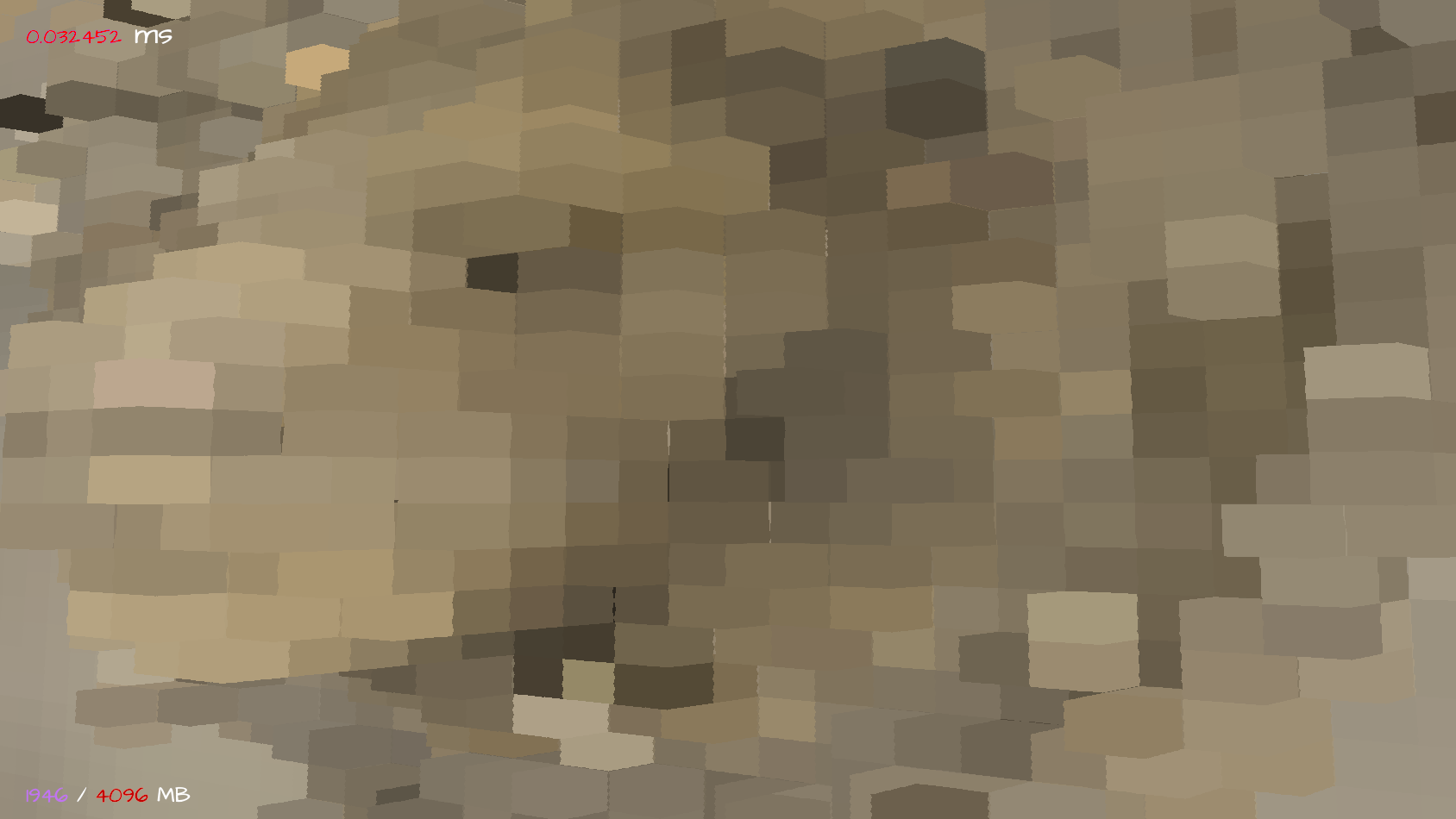To check which voxels should be generated the world-space location of the triangle in each of the four corners of the fragment pixel can be computed using the partial derivatives $\frac{\partial p}{\partial x}, \frac{\partial p}{\partial y}$ of the fragment world position vector $p$, exposed through dFdx and dFdy in the fragment program. Those points can be projected onto the projection direction (the triangle dominant axis) to check if additional voxels are overlapped.

p00 = p + .5 * (-dFdx(p) - dFdy(p));
p10 = p + .5 * ( dFdx(p) - dFdy(p));
p01 = p + .5 * (-dFdx(p) + dFdy(p));
p11 = p + .5 * ( dFdx(p) + dFdy(p));


# Conflict Resolution

We need a way to handle overlapping voxels from different voxel fragments. Overwriting the same coordinate in the grid with different colors would generate race conditions resulting in visible flicker. Instead we want to use hardware atomic operations, specifically an atomicAdd which can be tens of times faster than doing it manually with an compare-exchange operation. At the moment this means using a vendor specific extension (NV_shader_atomic_fp16_vector) and f16 data format. Till the OpenGL atomics specification matures a compare-exchange can be used as a working alternative for other vendors and/or image formats.

We use 2 3D images, one exclusively for color and one for normal + counter. This allows us to have full 4-channel color data. The images are cleared before each frame with glClearTexImage calls (GL_ARB_clear_texture, core since 4.4), the voxels are merged as follows.

s = voxel_size(p);
coords = round(P / s) + imageSize(voxel_radiance[level]) / 2;

imageAtomicAdd(voxel_normals[level], coords, f16vec4(n * coverage, coverage));


Reading voxels is a straightforward process, the values need to normalized by the w component of the normal image.

# Generating the Octree

The second pass is done in a compute shader that upsamples the layers to generate an octree. Special care needs to be taken to upsample only the commited parts of each level. Also due to our data structure, hardware linear filtering can not be used, therefore the 8 texels need to be fetched and added manually.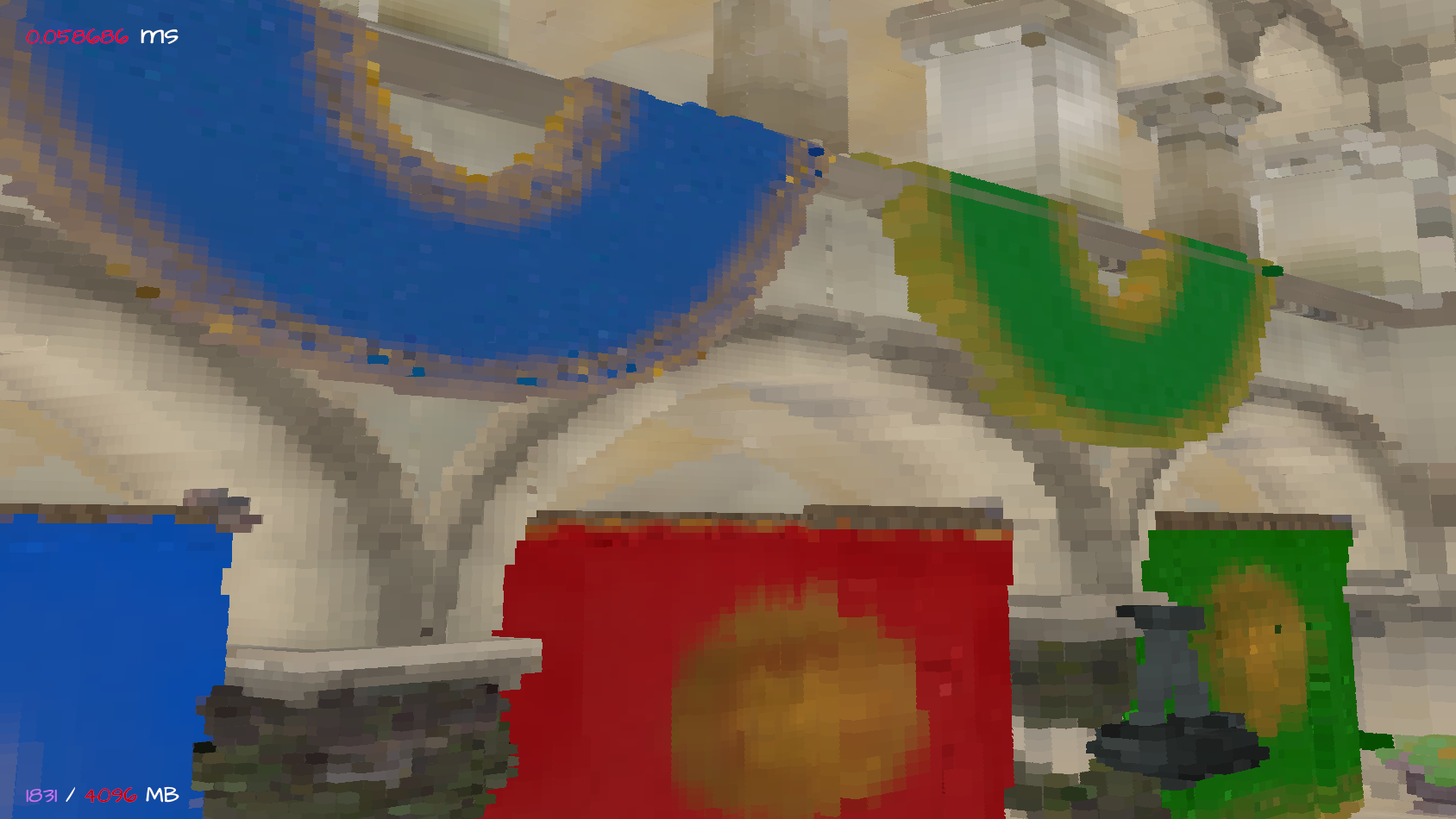# Summary and Results

The approach detailed above provides 6-separating planes voxelization with good performance, allowing real-time voxelization of the entire scene with good resolution and acceptable memory footprint.

Because the center of the voxel grid depends on the camera location, temporal artifacts occur during camera movements, i.e. swimming colors as voxels move and the popping of voxels that cross mipmap boundaries. To minimize that to an acceptable level the movement of the voxel grid should be discrete at jumps the size of a few voxels.

A more sophisticated implementation would calculate triangle coverage of each voxel. At the moment I only do it when voxelizing in the geometry shader, where the coverage is estimated by the triangle area over voxel size squared. Calculating coverage of voxel fragments is trickier and might be too expensive, a valid approach might include multisampling and counting the samples per voxel fragment.

Performance measurements were carried out on an nVidia GTX 980.

(The frame times in the screenshots above should be ignored, as they are affected mainly by the very naïve raytracing that renders the voxel world)

| Voxel grid size    | Sparse images size (2 X RGBA16F) | Render time (clear, voxelize, upsample) |
| ------------------ | -------------------------------- | --------------------------------------- |
| $1024^3$, 8 steps  | ~900MB                           | ~13ms (4.5ms, 4ms, 5ms)                 |
| $512^3$, 2 steps   | ~2500MB                          | ~30ms (12ms, 5ms, 13ms)                 |
| $512^3$, 4 steps   | ~640MB                           | ~8.5ms (3ms, 3ms, 2.5ms)                |
| $512^3$, 8 steps   | <100MB                           | ~3ms (0.5ms, 2ms, 0.5ms)                |


Clearing and upsampling is directly related to the voxel space size and is very bandwidth limited. The voxelization time isn’t affected much by the images’ size, as the bottleneck are the atomic operations. Ironically, the smaller the voxel grid, the more conflicts we have, therefore higher atomic overhead.